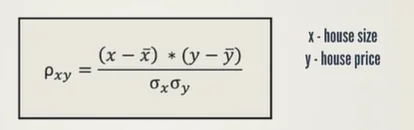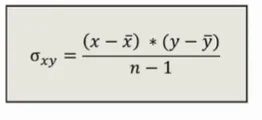21 Jul 2023

Posted on:

19 Jul 2023

0

# why there is difference between the formula of covar.

hello,

may i know why the formula of the formula is different, or the two formula is same

really i don't understand, so please sir provide to me a better explanation to this case.Instructor
Posted on:

21 Jul 2023

0

Hi Amal!

Thanks for reaching out.

In the first formula, we are calculating the correlation. It regards an estimate of the behaviour in a population. The notation we use is the greek letter pronounced 'rho'.

The second formula is about estimating covariance. It relates to sample data. The notation we use is the greek letter pronounced 'sigma'.

If we have all the data at hand, we can use the first formula to calculate the covariance between two stocks/securities. If we didn't, we'd need to use the second formula to compute the variance between two stocks in a sample.

Hope this helps.
Kind regards,
Martin